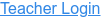• Ohm's Law
• Circuits
• Current
• Resistance
• Voltage

### Description

See how the equation form of Ohm's law relates to a simple circuit. Adjust the voltage and resistance, and see the current change according to Ohm's law.

### Sample Learning Goals

• Predict how current will change when resistance of the circuit is fixed and voltage is varied.
• Predict how current will change when voltage of the circuit is fixed and resistance is varied.
Version 1.4.9